Next: Capillary Length Up: Surface Tension Previous: Young-Laplace Equation

# Spherical Interfaces

Generally speaking, the equilibrium shape of an interface between two immiscible fluids is determined by solving the force balance equation (2.1) in each fluid, and then applying the Young-Laplace equation to the interface. However, in situations in which a mass of one fluid is completely immersed in a second fluid--for example, a mist droplet in air, or a gas bubble in water--the shape of the interface is fairly obvious. Provided that either the size of the droplet or bubble, or the difference in densities on the two sides of the interface, is sufficiently small, we can safely ignore the effect of gravity. This implies that the pressure is uniform in each fluid, and consequently that the pressure jump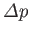is constant over the interface. Hence, from Equation (3.12), the mean curvatureof the interface is also constant. Because a sphere is the only closed surface which possesses a constant mean curvature, we conclude that the interface is spherical. This result also follows from the argument that a stable equilibrium state is one which minimizes the free energy of the interface, subject to the constraint that the enclosed volume be constant. Thus, the equilibrium shape of the interface is that which has the least surface area for a given volume: in other words, a sphere.

Suppose that the interface corresponds to the spherical surface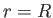, whereis a spherical coordinate. (See Section C.4.) It follows that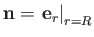. (Note, for future reference, that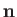points away from the center of curvature of the interface.) Hence, from Equation (C.65),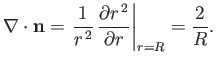(3.13)

The Young-Laplace equation, (3.12), then gives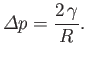(3.14)

Thus, given thatis the pressure jump seen crossing the interface in the opposite direction to, we conclude that the pressure inside a droplet or bubble exceeds that outside by an amount proportional to the surface tension, and inversely proportional to the droplet or bubble radius. This explains why small bubbles are louder that large ones when they burst at a free surface: for instance, champagne fizzes louder than beer. Note that soap bubbles in air have two interfaces defining the inner and outer extents of the soap film. Consequently, the net pressure difference is twice that across a single interface.Next: Capillary Length Up: Surface Tension Previous: Young-Laplace Equation
Richard Fitzpatrick 2016-01-22